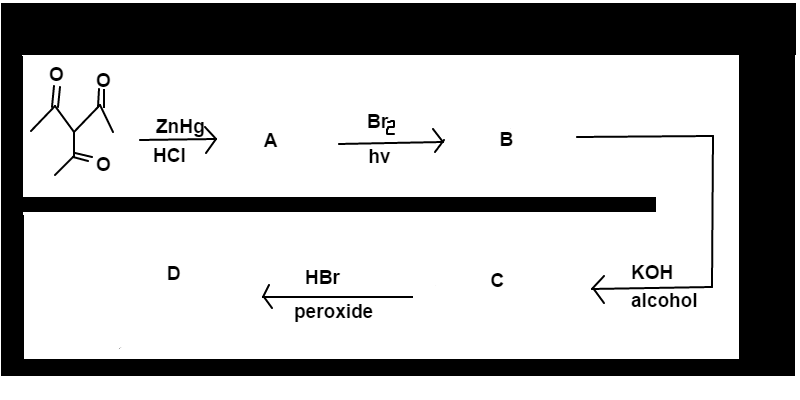# Bromine Hopping

Chemistry Level 2Starting with 3-acetyl-2,4-pentadione, precede through the reactions shown above. $B$ and $D$ will both be products that have the standard name: $\boxed{Number}$-bromo-3-ethyl pentane. You must determine which carbon ($c$) positions the Bromine will be in products $B$ and $D$. Find the difference of those positions going from product $B$ to $D$.

For example, if the Bromine is on $c_5$ of product $B$ and $c_1$ of product $D$ then your answer will be $5 - 1 = 4$.

Use IUPAC numbering protocol when answering this question.

David's Organic Chemistry Set

David's Physical Chemistry Set

×

Problem Loading...

Note Loading...

Set Loading...